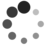# “茴”字有几种写法：系统负载是怎样计算的？（三）

By Chen Jie of TinyLab.org 2017-07-23 00:00:00

## 前情回顾

• 负载 == “采样时，D + R 的任务总数”
• 采样周期 5s
• 采样那会 的最近 1 分钟 / 5 分钟 / 10 分钟均值
• 1 分钟：`历史值 * e^(-5/60) + 当前值 * (1.0 - e^(-5/60))`
• 5 分钟：`历史值 * e^(-5/300) + 当前值 * (1.0 - e^(-5/300))`
• 15 分钟：`历史值 * e^(-5/900) + 当前值 * (1.0 - e^(-5/900))`

• 加权平均负载，采样周期 1ms：
• 周期内：累加 “R 状态的任务”时长 * 任务优先级 * CPU 频率缩放系数
• 多周期平均：a + a1 * q + a2 * q^2 …; // aN 代表距今前 N 个周期，q^32 = 0.5
• CPU 虚拟算力，固有算力基础上，剔除 RT 开销。剔除方法：
• 累加 RT 时长 * CPU 频率缩放系数
• 每 500ms 为一周期
• 距今 N 周期，则 `累加值 >> N` （←_← 跨周期边界，跳崖式衰减；一次跨地越多，坠地越快）
• 计算 RT 时间占比
• 1.0 - RT 时间占比，是为折扣系数 —— 固有算力上打折，即为虚拟算力

1. 任务们在队列中呆了一段时间，便是负载（当前 CPU 的 TODO list）。计算时，负载已经随 CPU freq 缩放，那不错。但还要据任务的 weight 缩放，weight 定义为何？
2. 历史的负载值（非本周期），如何随时间衰减？

## 普通任务的 weight 定义

CFS 中，任务 nice 级别，最终换算成了权重（weight）。越“重”，则虚拟时间（vruntime）流逝越慢：

``````update_curr()
u64 delta_exec;
...
delta_exec = now - curr->exec_start;
curr->exec_start = now;
...

curr->vruntime += calc_delta_fair(delta_exec, curr);
``````

“越重时间流逝越慢”的效应，按照伪科学类比法，就好像相对论重力越强时间流逝越慢：• 对于 Wake up preempt：阀值计算依赖候选者的 weight，候选者“越重”，越容易抢占。
• 对于 Tick preempt：阀值计算依赖 current 的 weight，current “越重”，越不容易被抢占。

``````static u64 decay_load(u64 val, u64 n)
{
unsigned int rest_n;

// 换言之，每经过 32 个周期右移一位，64 位数则有：
//   超过 32*63 个周期后，衰减为 0（或确切地说，不足一位）
if (unlikely(n > LOAD_AVG_PERIOD * 63))
return 0;

// 转成 32bits
rest_n = n;

/*
* As q^PERIOD = 1/2, we can combine
*    q^n = 1/2^(n/PERIOD) * q^(n%PERIOD)
* With a look-up table which covers q^n (n<PERIOD)
*
* To achieve constant time decay_load.
*/
}

// val *= q^rest_n，其中 q^rest_n 查表得到，即 runnable_avg_yN_inv[rest_n]
// 表中值采用 32 位整数模拟小数，故而算完需 “shift right 32”
val = mul_u64_u32_shr(val, runnable_avg_yN_inv[rest_n], 32);
return val;
}
``````

``````# python2 -c "print 0.5 ** (1.0/32)"
# 0.978572062088
#
# python2 -c "print '0x%x' % int(  (0.5 ** (1.0/32)) ** 31 * (1<<32)  )"
# 0x82cd8698, 即 runnable_avg_yN_inv 的值
``````

``````LOAD = weight * freq_scale * decay
decay = q^0 + q^1 + q^2 + ... q^n
``````

decay 因子计算，对应代码为 `__update_load_avg() / __compute_runnable_contrib(n)`

``````static u32 __compute_runnable_contrib(u64 n)
{
u32 contrib1 = 0;
u32 contrib2 = 0;

return runnable_avg_yN_sum[n];

// __accumulated_sum_N32[] 为每隔 32 个周期的、一系列预先计算的、等比求和值

// runnable_avg_yN_sum[] 为 1-31 个预先计算的、等比求和值
contrib2 = runnable_avg_yN_sum[n];

return contrib1 + contrib2;
}
``````

``````# python -c "print 1/(1 - 0.5 ** (1.0/32))"
# 46.6680463651

# 10 位整数模拟小数
# python -c "print 1/(1 - 0.5 ** (1.0/32)) * 1024"
# 47788